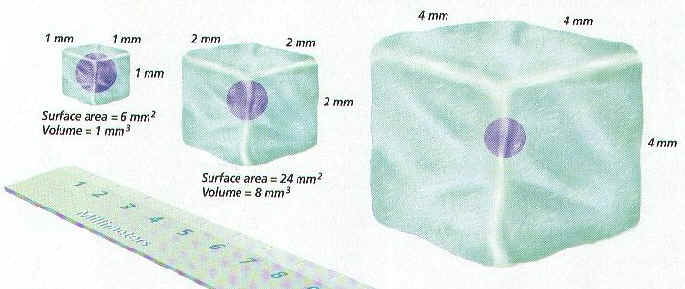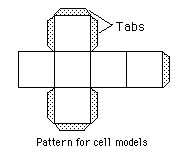# Cell Size

THE SURFACE AREA TO VOLUME RATIO OF A CELLINTRODUCTION:

Cells are limited in how large they can be. This is because the surface area and volume ratio does not stay the same as their size increases. Because of this, it is harder for a large cell to pass materials in and out of the membrane, and to move materials through the cell.
In this lab, you will make cube shaped models to represent cells. The dimension along one side will be doubled with each model. You will then calculate the surface area, volume, and the ratio between the two.MATERIALS:

Scissors
Construction paper
Tape
Metric ruler

PROCEDURE:

1. Construct three cell models like the pattern shown. The dimensions of a side will double each time, with the sides being 2 cm, 4 cm, and 8 cm. Fold and tape into cubes with the tabs to the inside. Record the dimensions in the DATA TABLE (the first one is done for you in the table).

## DATA TABLE: Cell Size Comparison

CellDimensions
(cm)
Surface Area
(cm2)
Volume
(cm3)
Ratio
Surface area to Volume
12 X 2 X 2
2
3

CALCULATIONS:

2. Calculate the total surface area for each cell model by the following formula:

surface area = (Length X Width) X 6 sides

Record the surface areas in the DATA TABLE.

3. Calculate the volumes for each cell model by the following formula:

volume = length X width X height

Record the volumes in the DATA TABLE.

4. Calculate the surface area-to-volume ratio for each cell model by the following formula:

ratio =surface area
volume

Record the ratio values in the DATA TABLE.
These ratios show how many times larger the surface area is as compared to the volume. Notice that it becomes less than one very quickly.

QUESTIONS:

1. Which model has the largest surface area?

2. Which model has the largest volume?

3. Which model has the largest ratio?

4. To maintain life, and carry-out cellular functions, materials must be able to move into and out of the cell. Also, material needs to be able to move within the cell. What might be the advantage of having a large surface area?

5. What might be the disadvantage of having a large volume?